# High School Math : Trigonometric Functions

## Example Questions

### Example Question #71 : Pre Calculus

Which of the following is not in the range of the function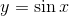?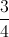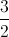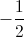Explanation:

The range of the functionis all numbers betweenand(the sine wave never goes above or below this).

Of the choices given,is greater thanand thus not in this range.

### Example Question #41 : Pre Calculus

Simplify the function below: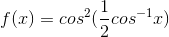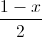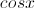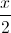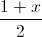Explanation:

We need to use the following formulas:

a)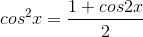and

b)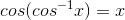We can simplifyas follows: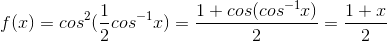### All High School Math Resources